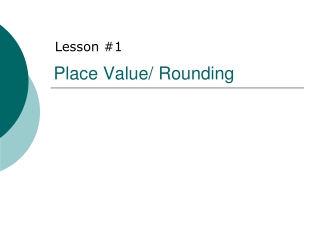DownloadDownload PresentationPlace Value/ Rounding

# Place Value/ Rounding

Download Presentation## Place Value/ Rounding

- - - - - - - - - - - - - - - - - - - - - - - - - - - E N D - - - - - - - - - - - - - - - - - - - - - - - - - - -
##### Presentation Transcript

1. Place Value/ Rounding Lesson #1

2. Reading and writing a decimal # 8.59 1122.0025

3. Standard Form • The full number • The way you “normally” write a number

4. What is the standard form of these expanded form numbers: 1) 800 + 40 + 3 = 2) 20 000 + 600 + 40 + 9 = 3) 1000x4 + 100x5 + 1x3 = 4) 100x9 + 1x8 + 1/10x5 =

5. Expanded Form • Writing a number showing the total value of each digit • Example: 543 100 x 5 = 500 10 x 4 = 40 1 x 3 = 3 So 543 = 500 + 40 + 3

6. Expanded Form: Practice • Complete the expanded form of the following numbers: 1) 384 = _______ + 80 + _______ 2) 6257 = 6000 + ________ + ______ + ______ 3) 37 = 10x3 + ________ 4) 4826 = ________ + 100x8 + _______ + _____ 5) 219.2 = ______ + _______ + ______ + ______

7. Exponential form

8. Exponential practice • Write the following numbers in exponential form. • 67.34 • 2 098 644

9. Rounding rules: • Find the number in the place value you are asked to round to (ones, tens, hundredths) • Look at the number to the right. • If its 4 or less round down. • If its 5 or more round up.

10. Examples • Nearest tens: 1265 • Nearest hundreds: 957 • Nearest thousandths .3472 • Nearest tenths .34